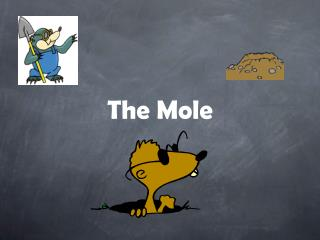# The Mole - PowerPoint PPT PresentationDownload PresentationThe Mole

The MoleDownload Presentation## The Mole

- - - - - - - - - - - - - - - - - - - - - - - - - - - E N D - - - - - - - - - - - - - - - - - - - - - - - - - - -
##### Presentation Transcript

1. The Mole

2. New Unit of Measurement : The Mole • 3 mathematical definitions:

3. New Measurement Unit: The Mole1st Definition • 1 mol= atomic mass of atom = molecular mass of a molecule = formula mass of an ionic compound MOLAR MASS These values come from the periodic table. The atomic mass #’s are in grams/1 mol

4. Calculating Formula Molecular mass • Need periodic table (keep the decimal numbers) • Count the number of atoms in a substance and multiply it by it’s mass number then add. Ex 1) K (atom) 39.098 g = atomic mass 1 mol Ex 2) P (atom) 30.974 g = atomic mass 1 mol

5. Ex 3) Potassium Chloride - KCl(formula unit) 1 K x 39.098 = 39.098 1 Cl x 35.453 = 35.453 74.551 g/ mol Ex 4) Magnesium Chloride - MgCl2 1 Mg x 24.305 = 24.305 2 Cl x 35.453 = 70.906 95.211 g/mol Ex 5) Ammonium Carbonate - (NH4)2CO3 2 N = 28.02 8 H = 8.08 1 C = 12.01 3O = 48.00 = 96.11 g/mol

6. New Measurement Unit: The Mole2nd and 3rd Definition • 2) 1 mol= 6.02 x 1023 particles (avagadro’s number) where a “particle” can be: a) molecule b) atom c) ion d) formula unit of ionic compound 3) 1 mol= 22.4 L for gas molecules

7. Volume of gas at STP formula mass, atomic mass, or molecular mass 22.4 dm3 / mole at STP (gases only) Mass Moles 6.02 x 1023 particles/ mole (Avogadro Number) number of particles (atoms, ions, molecules, formula units)

8. One-Step Conversion Problems • Use road maps to convert between one unit of chemical quantity to another. • Dimensional Analysis – like metrics • Use the three definitions of a mole; an extension of the definitions in numerical form.

9. One-Step Conversion Problems • These are your conversions factors! 1) 1 mole or x grams x grams 1 mole • 2) 1 mol or 6.02 x 1023 particles • 6.02 x 1023 particles 1 mole • 3) Gases ONLY!1 mol or 22.4 L • 22.4L 1 mol

10. One- Step Conversions Ex 1) Convert 4.3 grams of NaCl to moles. Mass  mol Given: Unk: 4.3 g NaCl x 1 mol NaCl= 7.4 x 10-2 mol NaCl 58.45 g NaCl Ex 2) Convert 0.00563 mol NH3 to grams. Mol -> massG: U: 0.00563 mol NH3 x 17 g NH3 =9.57 x 10 –2 g NH31 mol NH3

11. More One-Steppers Ex) Given: 0.91 Mol NaClO3 Unk: ? Formula Units NaClO3 Ex) Given 22.92 x 1018molecules Cl2 Unk: ? Mol Cl2

12. More One-Steppers Ex) Given: 460. Mol Cl2gas Unk: ? L Cl2 Ex) Given 84.56 L Cl2 Unk: ? Mol Cl2

13. TWO –STEP CONVERSIONS Ex 1) Given: 8.631 x 1021 atoms Na Unkn: ? g Na

14. TWO –STEP CONVERSIONS Ex 2) Given: 1.5 x 1022f.u. MgCl2 Unkn: ? g MgCl2

15. TWO –STEP CONVERSIONS Ex 3) Given: 2.63 L O2 Unkn: ? mg O2

16. % Composition • Definition: the % (in mass) of each element in a compound % mass element = g element x 100% g compound Ex An 8.40 g sample of flourine completely combines with a 4.90 g sample of sodium. Calculate the % composition of the compound that forms. mass element 1 + mass element 2 = total; 8.40 g F2 + 4.90 g Na = 13.30 g NaF %F: 8.40 g x 100 = 63.2% F 13.30 g %Na: 4.90g x 100 = 36.8% Na 13.30 g

17. % mass CuSO4 1 Cu x 63.546 g = 63.546 g 1 S x 32.066 g = 32.066 g 4 O x 15.999 g = 63.996 g x 159.608 g % Cu= 63.546 x 100 = 39.8% 159.608 % S= 32.066 x 100 = 20.1% 159.608 %O= 63.996 x 100 = 40.1% 159.608

18. HYDRATES • Compounds with water molecules chemically attached to ionic crystalline structure formula – CuSO4 5H2O name – copper (II) sulfate pentahydrate CaSO44H2O - BaCl2 9H2O - H2O molecules can be removed by heating the compound. This is not evaporation. Therefore, it is NOT a physical change. (Decomposition reaction)

19. Mole-to-Mole Relationships • Decomposition of water: 2H20 2H2 + O2 2 mol of H20 yields 2 mol H2 and 1 mol O2 Ex) You have 4 mol of water. If you decompose 4 mol of water, how many mols of products do you get? 2[2H2O 2H2 + O2] 4H2O 4H2 + 2O2 4 mol of H2O yields 4 mol of H2 plus 2 mol of O2